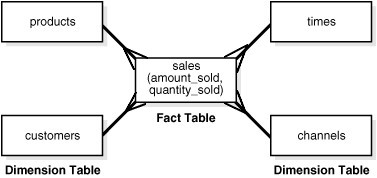# Dimensional Data Modeling - OLAP Cube

An OLAP cube is a dimensional data structure that answer dimensional query. It store data in a multidimensional way. You can a cube as an extension of the two dimensions relational table.

## Creation

A cube is defined:

• its dimension (and hierarchy)
• and measures (calculation)

and is generally pre-computed.

## Computation

The OLAP cube consists of numeric facts called measures which are categorized by dimensions. The cube metadata may be created from a star schema or snowflake_schema of tables in a relational database. Measures are derived from the records in the fact table and dimensions are derived from the dimension tables.

Discover MoreDimensional Data Modeling - Dimensional Schemas

This section is dimensional data modeling, That is the building of a cube (hypercube) A dimensional schema is a schema based on dimension. The dimensional schema can modeled: in a relational database...Dimensional Data Operation - Drill through

Drill through is a dimensional data operation that describe the act of exploring or browsing items like folders, files or related components. Generally associated with drill down and drill up, which...Multidimensional Data (Cube)

A cube can be thought of as extensions to the two-dimensional array of a spreadsheet. A cube is also known as: a multidimensional cube or a hypercube It consists of numeric facts called measures...